# Chapter 5. Introduction of Western Mathematics

## Column Transcendental Equation (Level 2)

Equations that do not fall in the category of algebraic equations are called transcendental equations.

So, what is the algebraic equation? It is an equation in the form “polynomial expression=0,”but any identity such as (x+1)2-(x2+2x+1)=0 is not called an equation. The equation x2+y2=1 is an algebraic equation, and its solution is not found in the range of natural numbers, and in the range of integers, x=±1, y=0 or x=0, y=±1 is the solution. In the range of rational numbers, if we let (a,b,c) be a Pythagorean triple satisfying a2+b2=c2, we have an infinite number of instances of the solution because x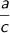, y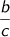is the solution. In the range of real numbers, if we assume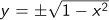for any x in the range of -1≦x≦1, we also have an infinite number of instances of the solution because x, y is the solution. When we try to find integer solutions to an equation having integer coefficients and multiple variables, the equation is called a Diophantine equation. The Fermat conjecture in the seventeenth century, stating that x=y=z=0 is the only solution to xn+yn=zn,in which n is a natural number greater than 3, was finally proved in 1995.

If a number (real number or complex number) is the solution to one algebraic equation having rational number coefficients and one variable, the number is called an algebraic number. For example, assuming the number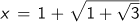, and it follows that (x-1)2=1+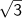and then x2-2x=. Next, raise both sides to the second power and we obtain x4-4x3+4x2-3=0. This means that x is an algebraic number, because it is the solution to x4-4x3+4x2-3=0. The theorem that the solution to an algebraic equation whose coefficients are complex numbers always falls in the range of complex numbers is called the fundamental theorem of algebra, and was proved by F. Gauss in 1799.

Then, if we concluded that all real numbers or complex numbers were algebraic numbers, we would be wrong. There are no algebraic equations to which the circular constant Pi (π) is the solution. Pi is the solution to an equation such as sin x=0 that is not an algebraic equation; real numbers like this that are not algebraic numbers are called transcendental numbers. Another famous transcendental number is the number e, known as the base of natural logarithms; if we produce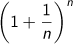for the natural number n, and increase n to infinity, we obtain the number 2.718281828…, which is e. When we form the function y=ex, it has a mysterious feature: however times we may differentiate it with respect to x, we obtain the same original function like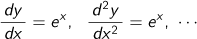,and so on. When we expand ex as a Taylor series, it can be written in a neat form as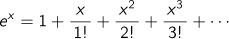.

It is known that the mysterious expression e+1=0 holds true, using this e and π.

G. Cantor (1845-1918) proved in 1874 that there are more transcendental numbers than algebraic numbers, but we do not yet know whether, for example, e+π or e×π is a transcendental number. Known specific transcendental numbers are limited.

Transcendental equations take a general form of expression, including exponential functions, logarithmic functions, and trigonometric functions, and normally assume a numerical solution.

Top of page